Courses

# Tunneling through a Barrier(Square and Step Barriers) - The Schrodinger Equation, Quantum Mechanics, Physics Notes | EduRev

## Physics for IIT JAM, UGC - NET, CSIR NET

Created by: Akhilesh Thakur

## Physics : Tunneling through a Barrier(Square and Step Barriers) - The Schrodinger Equation, Quantum Mechanics, Physics Notes | EduRev

The document Tunneling through a Barrier(Square and Step Barriers) - The Schrodinger Equation, Quantum Mechanics, Physics Notes | EduRev is a part of the Physics Course Physics for IIT JAM, UGC - NET, CSIR NET.
All you need of Physics at this link: Physics

The square barrier:

Behaviour of a classical ball rolling towards a hill (potential barrier):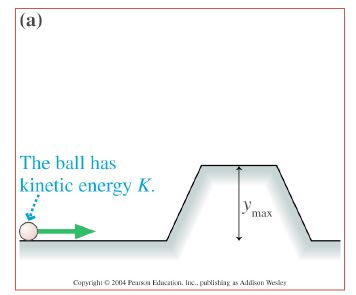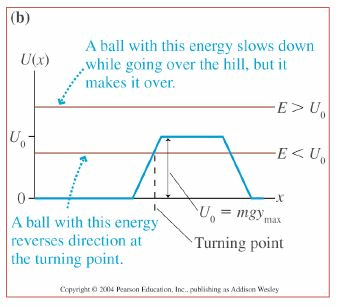If the ball has energy E less than the potential energy barrier (U=mgy), then it will not get over the hill.
The other side of the hill is a classically forbidden region.

Behaviour of a quantum particle at a potential barrier

Solving the TISE for the square barrier problem yields a peculiar result: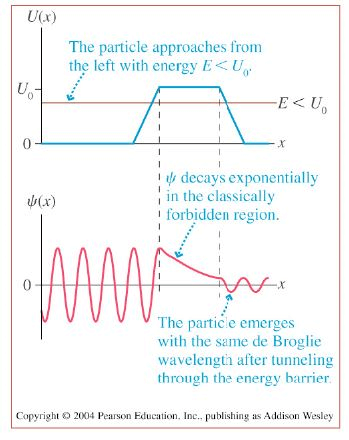If the quantum particle has energy E less than the potential energy barrier U, there is still a non-zero probability of ﬁnding the particle classically forbidden region !
This phenomenon is called tunneling.

Behaviour of a quantum particle at a potential barrier

To the left of the barrier (region I), U=0 Solutions are free particle plane waves: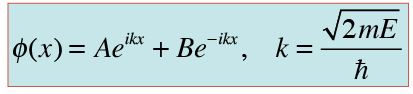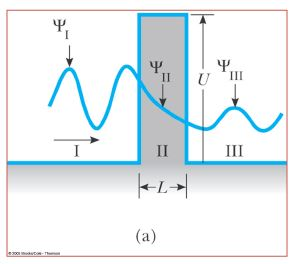The ﬁrst term is the incident wave moving to the right The second term is the reﬂected wave moving to the left.

Reﬂection coefﬁcient: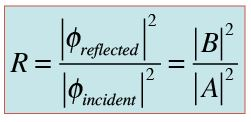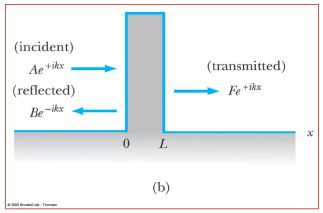Behaviour of a quantum particle at a potential barrier

To the right of the barrier (region III), U=0. Solutions are free particle plane waves: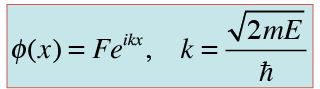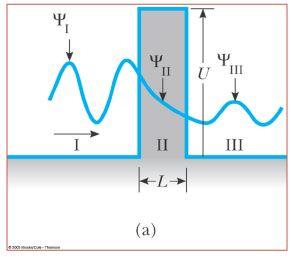This is the transmitted wave moving to the right

Transmission coefﬁcient: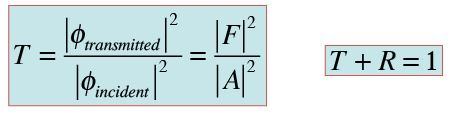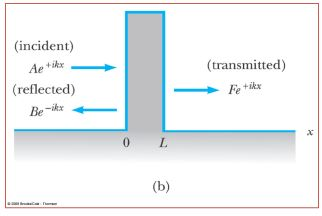Behaviour of a quantum particle at a potential barrier

In the barrier region (region II), the TISE is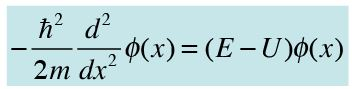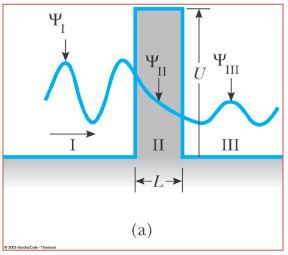Solutions are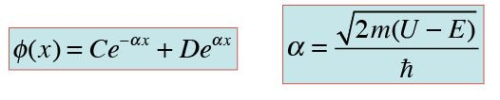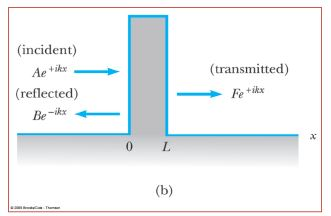Behaviour of a quantum particle at a potential barrier

At x=0, region I wave function = region II wave function: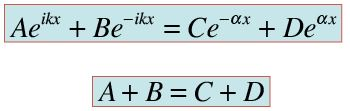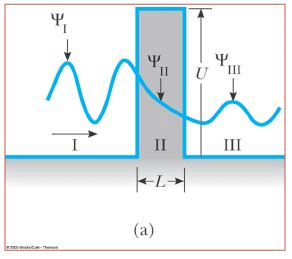At x=L, region II wave function = region III wave function: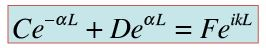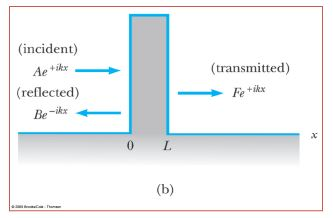Behaviour of a quantum particle at a potential barrier

At x=0, dϕ/dx in region I = dϕ/dx in region II:

At x=L, dϕ/dx in region II = dϕ/dx in region III :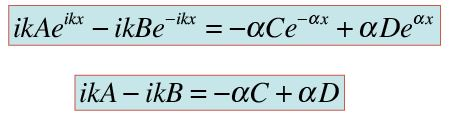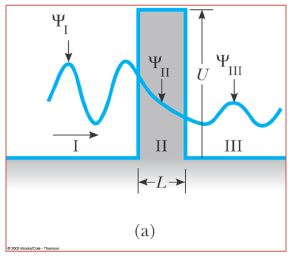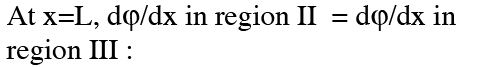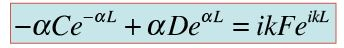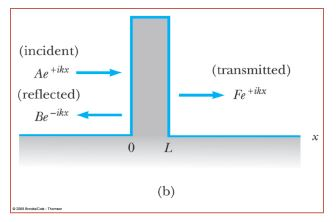Behaviour of a quantum particle at a potential barrier

Solving the 4 equations, we get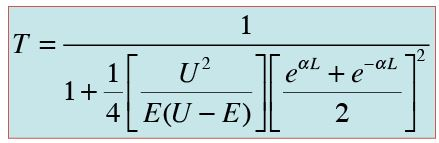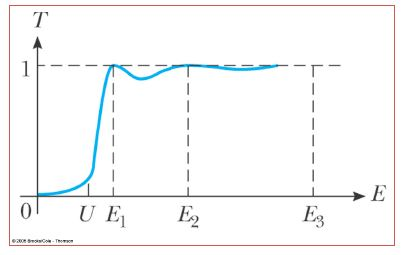For low energies and wide barriers,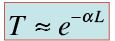For some energies, T=1, so the wave function is fully transmitted (transmission resonances).
This occurs due to wave interference, so that the reﬂected wave function is completely suppressed.

The step barrier:

To the left of the barrier (region I), U=0.
Solutions are free particle plane waves: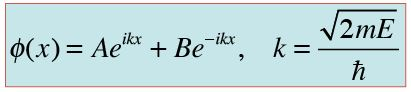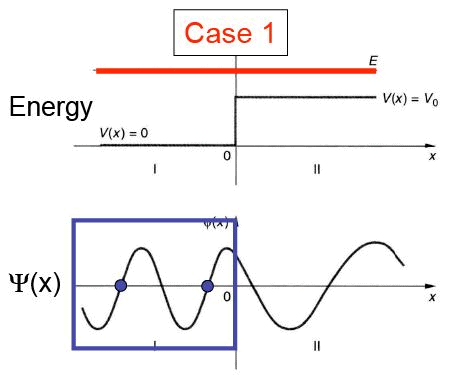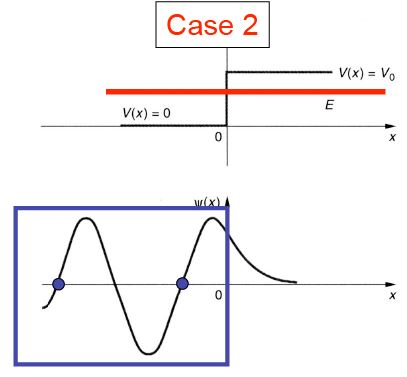Inside Step:
U = Vo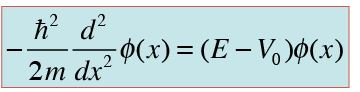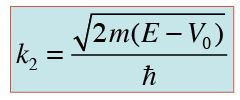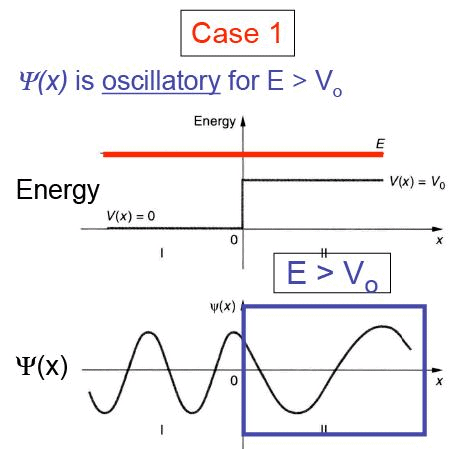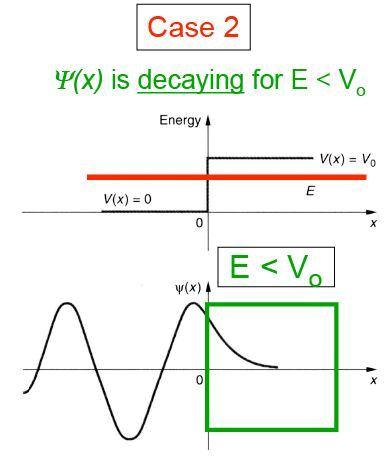The step barrier: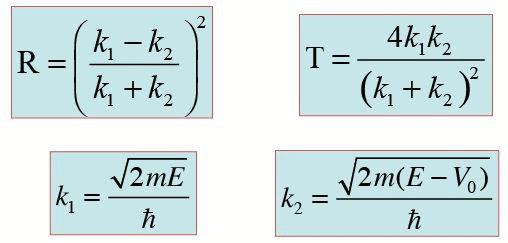R(reflection) + T(transmission) = 1

Reflection occurs at a barrier (R ≠ 0), regardless if it is step-down or step-up.
R depends on the wave vector difference (k1 - k2) (or energy difference), but not on which is larger.
Classically, R = 0 for energy E larger than potential barrier (Vo).

A free particle of mass m, wave number k1 , and energy E = 2Vo is traveling to the right. At x = 0, the potential jumps from zero to –Vo and remains at this value for positive x. Find the wavenumber k2 in the region x > 0 in terms of k1 and Vo. Find the reflection and transmission coefficients R and T.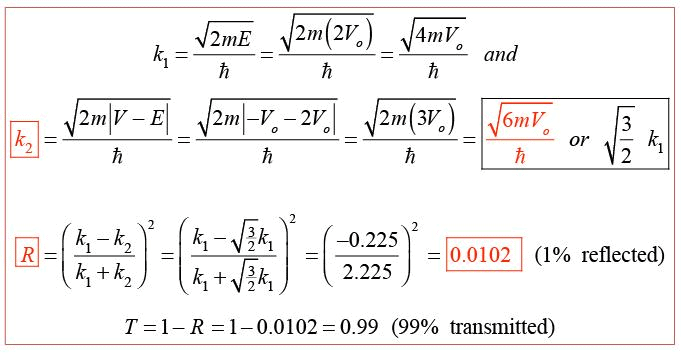Sketch the wave function ψ(x) corresponding to a particle with energy E in the potential well shown below. Explain how and why the wavelengths and amplitudes of ψ(x) are different in regions 1 and 2.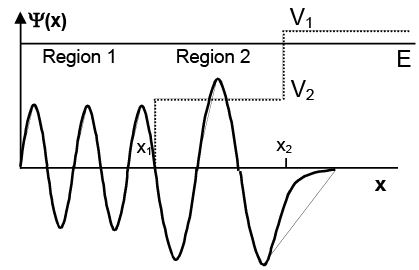ψ(x) oscillates inside the potential well because E > V(x), and decays exponentially outside the well because E < V(x).
The frequency of ψ(x) is higher in Region 1 vs. Region 2 because the kinetic energy is higher [Ek = E - V(x)].
The amplitude of ψ(x) is lower in Region 1 because its higher Ek gives a higher velocity, and the particle therefore spends less time in that region.

Sketch the wave function ψ(x) corresponding to a particle with energy E in the potential shown below. Explain how and why the wavelengths and amplitudes of ψ(x) are different in regions 1 and 3.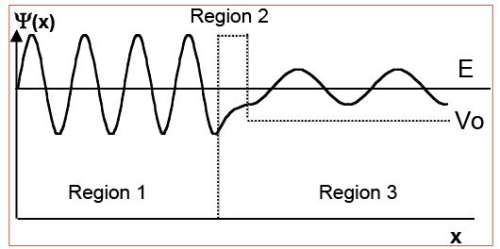ψ(x) oscillates in regions 1 and 3 because E > V(x), and decays exponentially in region 2 because E < V(x).
Frequency of ψ(x) is higher in Region 1 vs. 3 because kinetic energy is higher there.
Amplitude of ψ(x) in Regions 1 and 3 depends on the initial location of the wave packet. If we assume a bound particle in Region 1, then the amplitude is higher there and decays into Region 3 (case shown above).

159 docs

,

,

,

,

,

,

,

,

,

,

,

,

,

,

,

,

,

,

,

,

,

,

,

,

,

,

,

;